Next: The Kerr metric Up: The Theory of General Previous: The Theory of General

# The basic concepts

In the theory of general relativity, gravity is treated as a curvature of space-time instead of a force. A point in the 4-dimensional space-time is called an event. The trajectory of a body in space-time is called a world line.

The separation ds between to infinitely close events with coordinates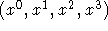and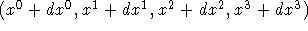, respectively, is given by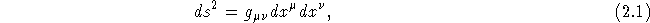where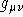is the metric tensor. We use the Einstein summation convention: repeated indices are to be summed over. If we wrote the above equation with explicit summation, it would be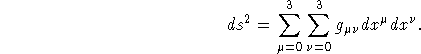We can chose any coordinates we like. For black holes, which a spherical symmetrical, time + spherical polar coordinates,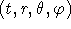, are well suited.

Web Exhibition: Null Geodesics Around a Kerr Black HoleBo Milvang-Jensen (milvang@astro.ku.dk)
Mon Jun 17 11:54:08 MDT 1996